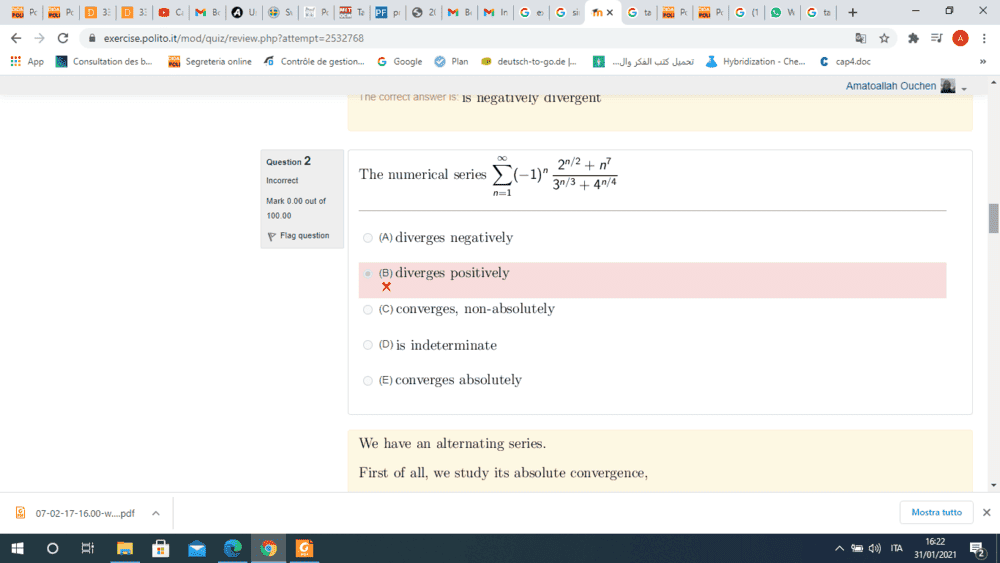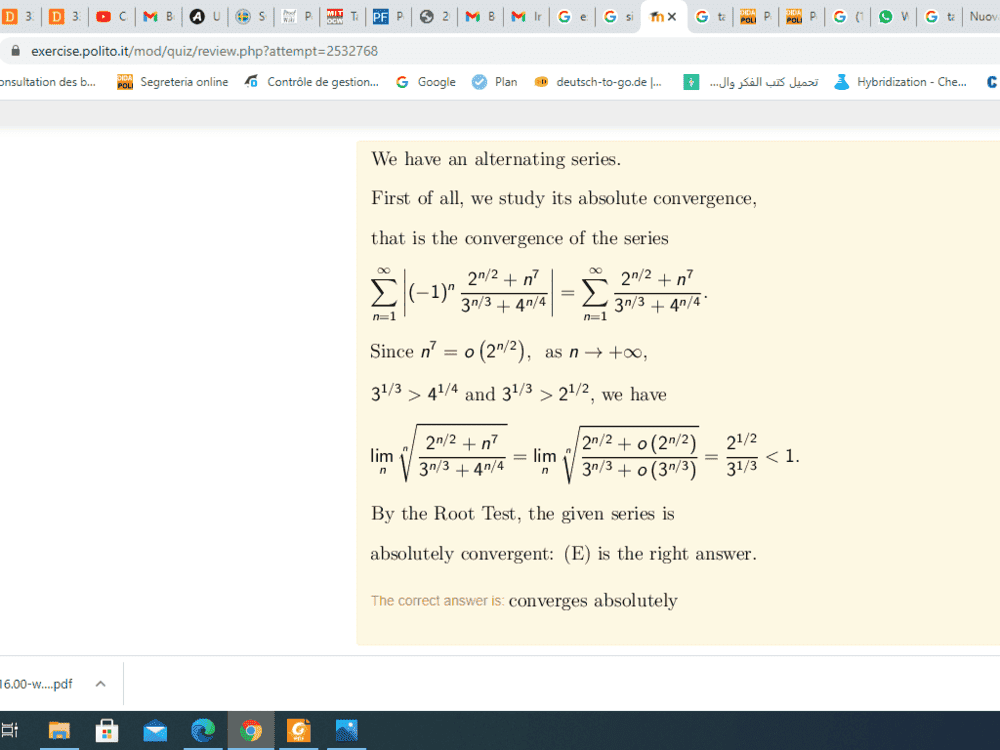# Problem with series convergence — Taylor expansion of exponential

• Amaelle

#### Amaelle

Homework Statement
problem with serie convergence (look at the image)
Relevant Equations
taylor serie expnasion, absolute convergence, racine test
Good dayand here is the solution, I have questions aboutI don't understand why when in the taylor expansion of exponential when x goes to infinity x^7 is little o of x ? I could undesrtand if -1<x<1 but not if x tends to infinity?

#### Attachments

Last edited by a moderator:
##n^7\neq o(n)##. I assume you meant the part where it says ##n^7=o(2^{n/2})##. Since ##\sqrt{2}>1##, ##2^{n/2}## dominates any power function as ##n\rightarrow\infty##.

•Amaelle
Sure. I assume you don’t understand why exponentials dominate power functions? Here is a simple proof: $$\log\frac{n^k}{b^n}=k\log(n)-n\log(b)$$ which tends to ##-\infty## as ##n\rightarrow\infty##, provided that ##\log(b)>0##. Thus, taking the exponential of both sides shows that $$\lim_{n\rightarrow\infty}\frac{n^k}{b^n}=\lim_{n\rightarrow\infty}e^{k\log(n)-n\log(b)}=0.$$
••